# RS Aggarwal Solutions for Class 8 Maths Chapter 5 - Playing with Numbers Exercise 5C

Students can download RS Aggarwal Solutions for Class 8 Maths Chapter 5- Exercise 5C, Playing with Numbers by referring to the links provided below. Our experts have solved the RS Aggarwal Solutions to help students in understanding the concepts. Practicing the solutions would definitely help in scoring good marks in their exams. In Exercise 5C of RS Aggarwal Class 8 Maths, we shall study Replacing alphabets by suitable numerals.

## Download PDF of RS Aggarwal Solutions for Class 8 Maths Chapter 5- Playing with Numbers Exercise 5C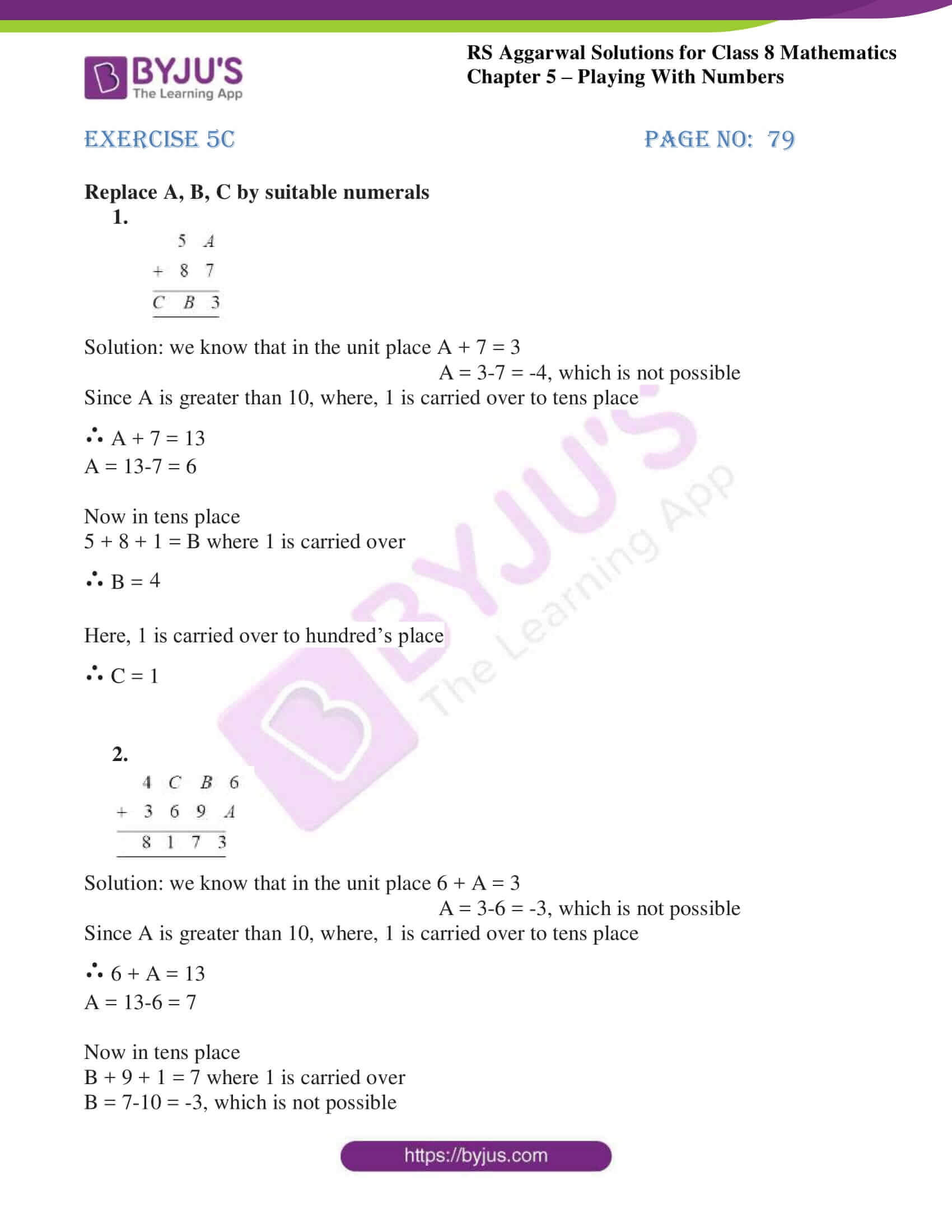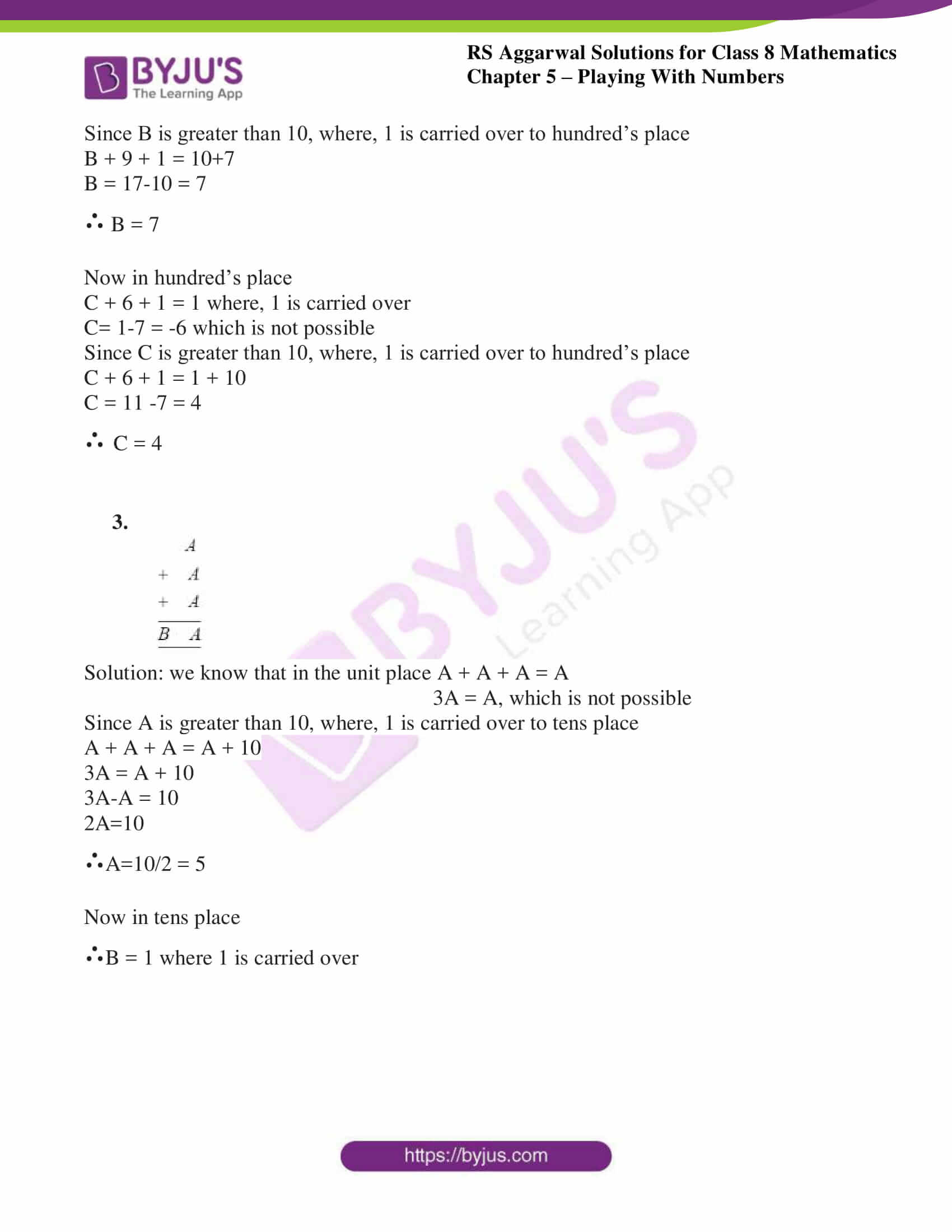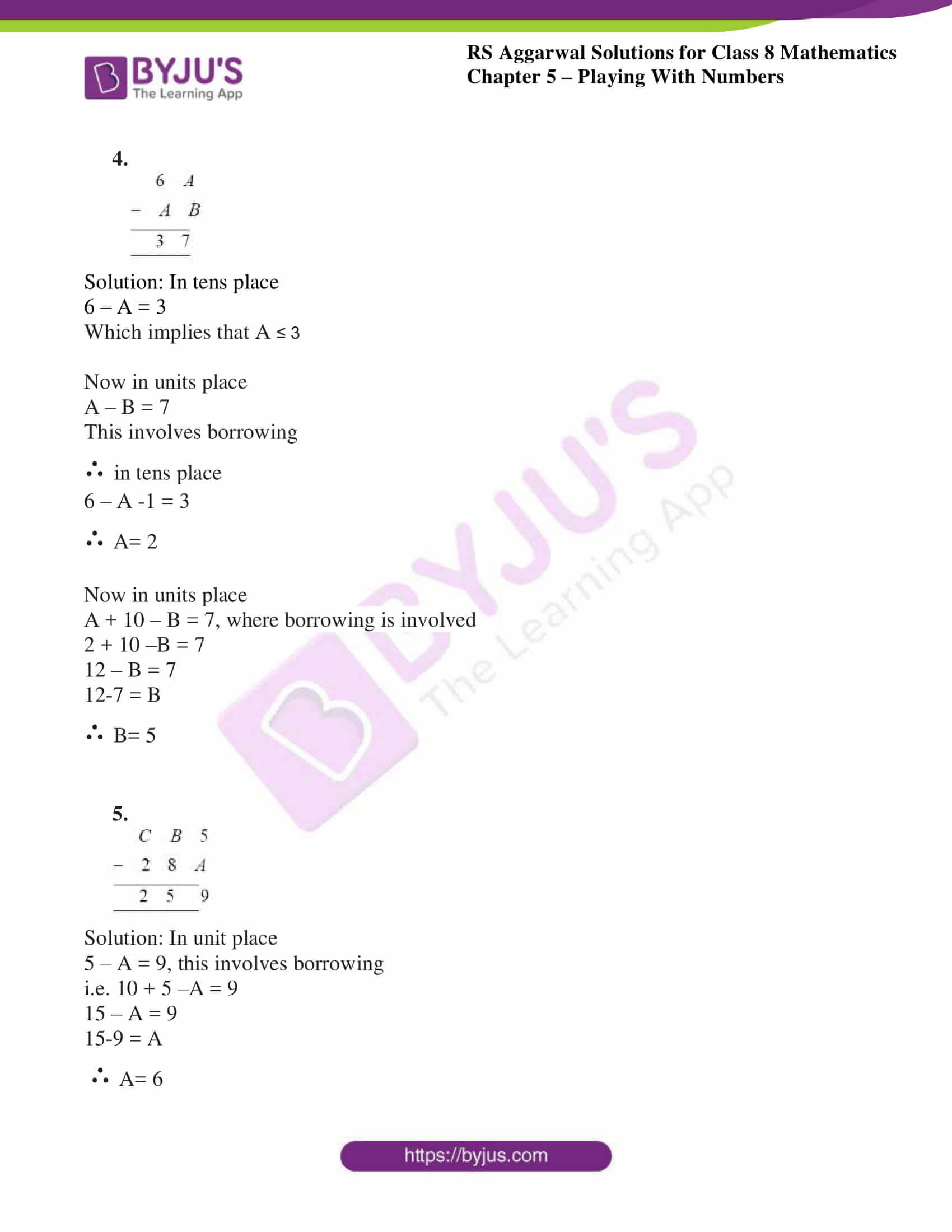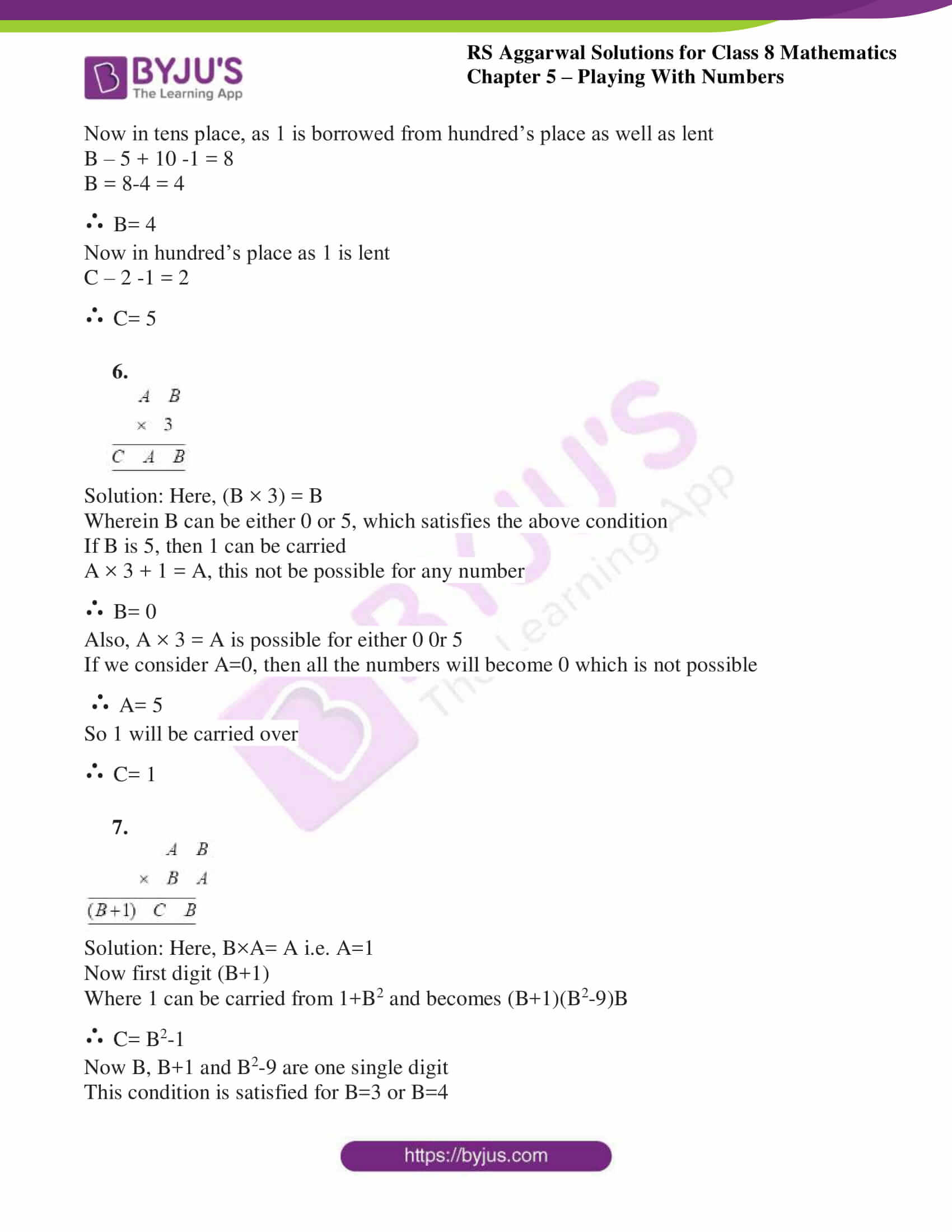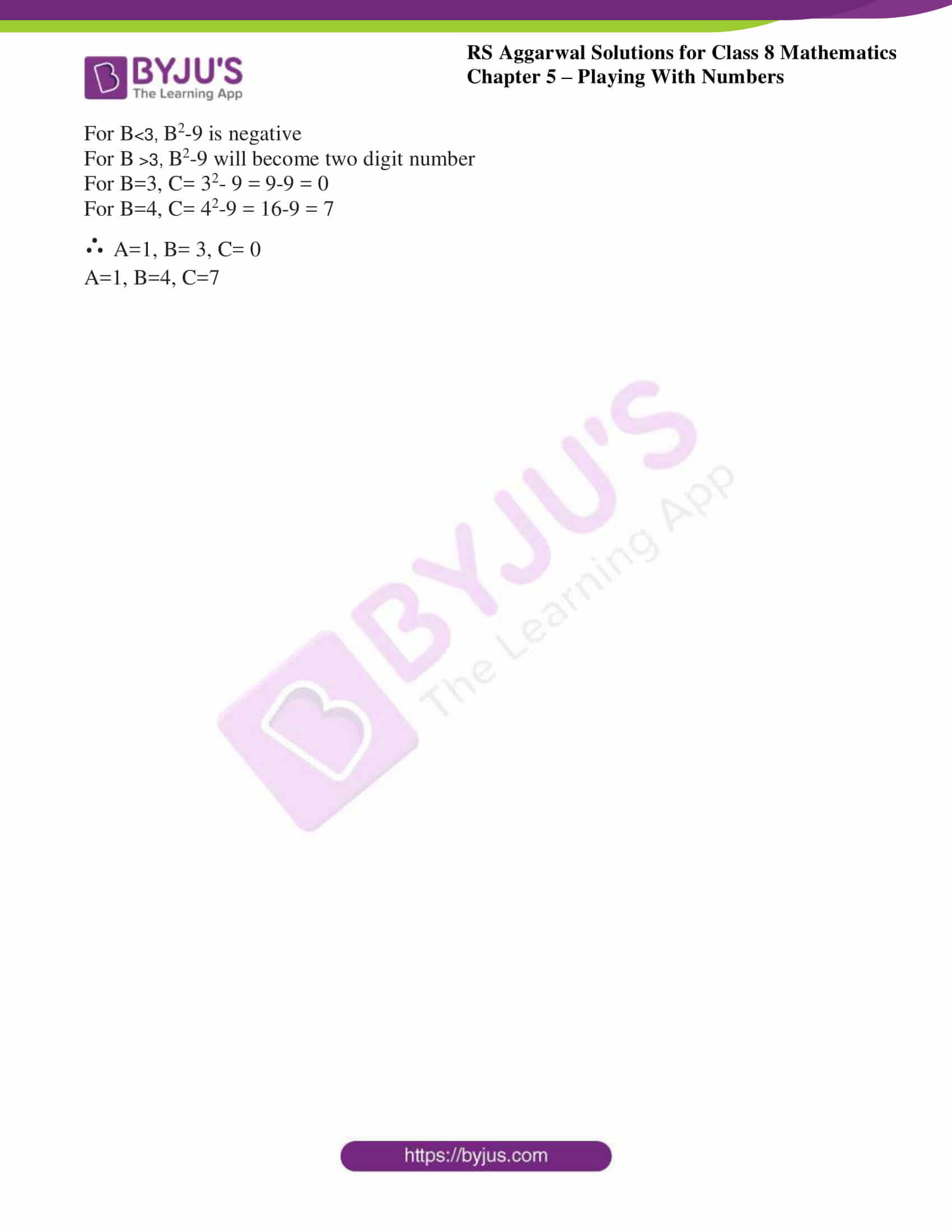### Access Answers to RS Aggarwal Solutions for Class 8 Maths Chapter 5- Playing with Numbers Exercise 5C

Replace A, B, C by suitable numerals

Q1.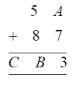Solution: we know that in the unit place A + 7 = 3

A = 3-7 = -4, which is not possible

Since A is greater than 10, where, 1 is carried over to tens place

∴ A + 7 = 13

A = 13-7 = 6

Now in tens place

5 + 8 + 1 = B where 1 is carried over

∴ B = 4

Here, 1 is carried over to hundred’s place

∴ C = 1

Q2.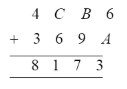Solution: we know that in the unit place 6 + A = 3

A = 3-6 = -3, which is not possible

Since A is greater than 10, where, 1 is carried over to tens place

∴ 6 + A = 13

A = 13-6 = 7

Now in tens place

B + 9 + 1 = 7 where 1 is carried over

B = 7-10 = -3, which is not possible

Since B is greater than 10, where, 1 is carried over to hundred’s place

B + 9 + 1 = 10+7

B = 17-10 = 7

∴ B = 7

Now in hundred’s place

C + 6 + 1 = 1 where, 1 is carried over

C= 1-7 = -6 which is not possible

Since C is greater than 10, where, 1 is carried over to hundred’s place

C + 6 + 1 = 1 + 10

C = 11 -7 = 4

∴ C = 4

Q3.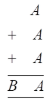Solution: we know that in the unit place A + A + A = A

3A = A, which is not possible

Since A is greater than 10, where, 1 is carried over to tens place

A + A + A = A + 10

3A = A + 10

3A-A = 10

2A=10

∴A=10/2 = 5

Now in tens place

∴B = 1 where 1 is carried over

Q4.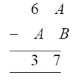Solution: In tens place

6 – A = 3

Which implies that A ≤ 3

Now in units place

A – B = 7

This involves borrowing

∴ in tens place

6 – A -1 = 3

∴ A= 2

Now in units place

A + 10 – B = 7, where borrowing is involved

2 + 10 –B = 7

12 – B = 7

12-7 = B

∴ B= 5

Q5.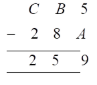Solution: In unit place

5 – A = 9, this involves borrowing

i.e. 10 + 5 –A = 9

15 – A = 9

15-9 = A

∴ A= 6

Now in tens place, as 1 is borrowed from hundred’s place as well as lent

B – 5 + 10 -1 = 8

B = 8-4 = 4

∴ B= 4

Now in hundred’s place as 1 is lent

C – 2 -1 = 2

∴ C= 5

Q6.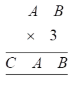Solution: Here, (B × 3) = B

Wherein B can be either 0 or 5, which satisfies the above condition

If B is 5, then 1 can be carried

A × 3 + 1 = A, this not be possible for any number

∴ B= 0

Also, A × 3 = A is possible for either 0 0r 5

If we consider A=0, then all the numbers will become 0 which is not possible

∴ A= 5

So 1 will be carried over

∴ C= 1

Q7.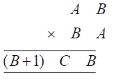Solution: Here, B×A= A i.e. A=1

Now first digit (B+1)

Where 1 can be carried from 1+B2 and becomes (B+1)(B2-9)B

∴ C= B2-1

Now B, B+1 and B2-9 are one single digit

This condition is satisfied for B=3 or B=4

For B<3, B2-9 is negative

For B >3, B2-9 will become two digit number

For B=3, C= 32– 9 = 9-9 = 0

For B=4, C= 42-9 = 16-9 = 7

∴ A=1, B= 3, C= 0

A=1, B=4, C=7

### Access other Exercises of RS Aggarwal Solutions for Class 8 Maths Chapter 5- Playing with Numbers

Exercise 5A Solutions 6 Questions

Exercise 5B Solutions 15 Questions

Exercise 5D Solutions 9 Questions

## RS Aggarwal Solutions for Class 8 Maths Chapter 5- Playing with Numbers Exercise 5C

Exercise 5C of RS Aggarwal Class 8, Playing with Numbers contains the concepts related to Numbers. This exercise mainly deals with the advanced concepts related to numbers which include replacing alphabets with suitable numerals.

The RS Aggarwal Solutions can help the students practice diligently while learning the fundamentals as it provides all the answers to the questions from the RS Aggarwal textbook. Students are suggested to practice the problems on a regular basis which will help them excel in their exams and increase their overall percentage. Practicing as many times as possible helps in upgrading time management skills and also boosts the confidence level to attain high marks.